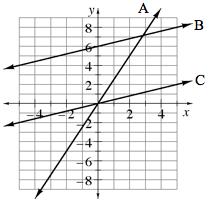### Home > MC2 > Chapter 7 > Lesson 7.1.4 > Problem7-37

7-37.

Compare the graphs of lines A, B, and C below.Which line increases most sharply?

Line A

2. What is the $y$-value of line A when $x = 2$?

Locate line A and $x = 2$ on the graph.

What is the $y$-coordinate of the point on the graph?

3. Compare the slopes of lines B and C.

The lines are parallel. Parallel lines have the same change in $y$-axis for the same change in $x$-axis.